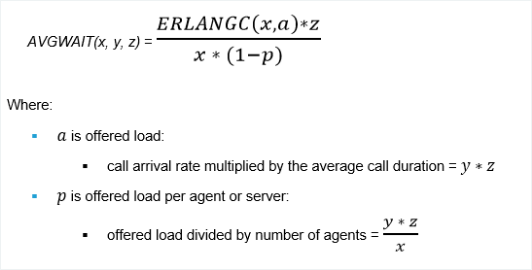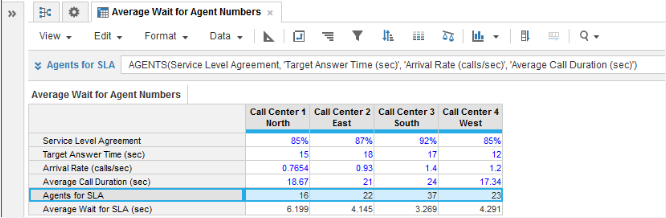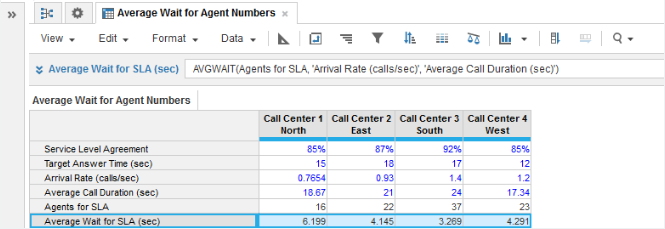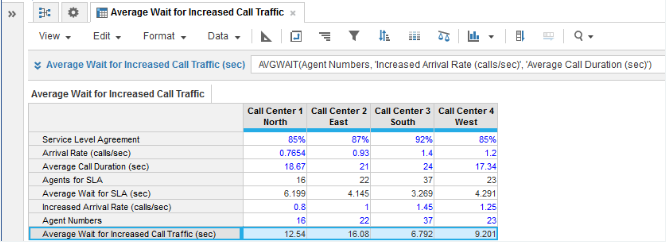1. Calculation functions
2. All Functions
3. Call Center Planning Functions
4. AVGWAITCalculates the average waiting time for incoming calls.

## Syntax

AVGWAIT(x, y, z)

where:

• x: number of servers, telephone lines, or agents
• y: arrival rate of calls or the number of calls per unit of time
• z: average call duration

## Format

Input Format Output Format

x: number

y: number (time unit)

z: number (time unit)

Number

## Arguments

The function uses the following arguments:

• x: Numeric line item, property, or expression
• y: Numeric line item, property, or expression - Must be the same time unit as used for argument z
• z: Numeric line item, property, or expression - Must be the same time unit as used for argument y

## Constraints

The function has the following constraints:

• All parameters are mandatory.
• All parameters must be a numeric line item, property, or expression.
• Time unit used for y and z must be the same.
• Result line item must be numeric.
• Maximum number of agents is five million.

Equation:

AVGWAIT is the solution to this equation:## Excel equivalent

• No Excel equivalent

## Example

In this example, we have four call centers each covering a separate geographical area:

• Each call center must achieve a service level agreement (SLA), expressed as the percentage of incoming calls that will be answered for a given target answer time.
• Each call center must achieve a target answer time for incoming calls.
• The arrival rate of incoming calls and the average call duration are known for each call center from historical monitoring of operational performance.

With the baseline metrics entered for each call center in numeric source line items for Service Level Agreement, Target Answer Time (sec), Arrival Rate (calls/sec), and Average Call Duration, we can first use the AGENTS function in an Agents for SLA numeric result line item to calculate how many agents are required for each call center to meet the SLA:We can then use AVGWAIT in a numeric result line item to calculate the average waiting time for incoming calls at each call center, when the number of agents available to receive calls is that required to maintain the SLA:Example 2

In a second example, we can perform what-if analysis for average waiting time for incoming calls at call centers. Suppose you want to determine what effect increased call traffic will have on the average waiting time at your call centers:

• The average duration of calls is known from historical monitoring of operational performance and is entered in the Average Call Duration (sec) numeric source line item.
• Agent staffing levels are known and entered using the Agent Numbers numeric source line item.
• The Increased Arrival Rate (calls/sec) numeric source line item is used to enter increased call traffic figures.

The Average Wait for Increased Call Traffic (sec) numeric result line item then provides the corresponding increased average call waiting times: